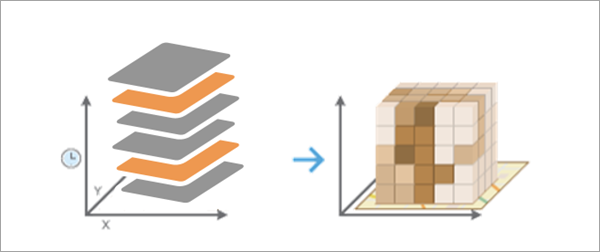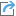# Create Space Time Cube From Multidimensional Raster Layer (Space Time Pattern Mining)

## Summary

Creates a space-time cube from a multidimensional raster layer and structures the data into space-time bins for efficient space-time analysis and visualization.

## Illustration## Usage

• The Output Space Time Cube can be analyzed for patterns in space and time using tools in the Space Time Pattern Mining toolbox, including Emerging Hot Spot Analysis, Local Outlier Analysis, and Time Series Clustering.

• The Output Space Time Cube will be created using the spatial and temporal resolution of the Input Multidimensional Raster Layer. Each space-time bin in the output cube will refer to a single raster cell for a single time interval from the input. Bins sharing the same location will have the same Location ID attribute, and bins sharing the same time interval will have the same Time Step ID attribute.

• This tool is similar to the Create Space Time Cube From Defined Locations and Create Space Time Cube By Aggregating Points tools, except no spatial or temporal aggregation is used in this tool for the conversion. The locations of the space-time cube are the same as the individual raster cells, and the time intervals of the cube are the same as the time intervals of the raster.

• Each location will be analyzed for trends in time using the Mann-Kendall statistic. This information, along with other properties of the space-time cube, is written as geoprocessing messages at the bottom of the Geoprocessing pane during tool execution. You can also access the messages using geoprocessing history by hovering over the progress bar and clicking the pop-out buttonor expanding the messages section in the Geoprocessing pane.

• You can visualize the space-time cube data in either 2D or 3D using the Visualize Space Time Cube in 2D or Visualize Space Time Cube in 3D tool, respectively, or by downloading the Space Time Cube Explorer add-in.

• This tool requires the Input Multidimensional Raster Layer be projected to accurately measure distances. If it is in a geographic coordinate system with latitude and longitude coordinates and no Output Coordinate System Environment setting is specified, the raster layer will be projected to the WGS 1984 World Equidistant Cylindrical projection (WKID 4087).

• The Input Multidimensional Raster Layer must have at least 10 time intervals to be used in this tool.

• Space-time cubes can store a maximum of two billion bins. If the number of raster cells multiplied by the number of time intervals exceeds two billion, the tool will return an error.

• Any raster cell that has NoData values for every time interval will be excluded from the output, and no space-time bin will be assigned.

• If your multidimensional raster is stored in Cloud Raster Format (*.crf), you can browse to or specify the file path of the raster dataset for the Input Multidimensional Raster Layer parameter without creating a multidimensional raster layer. If the raster is multivariate, the first variable will be used by the tool. To use a different variable, you must add the variable as a multidimensional raster layer using the Import Variables From Multidimensional Raster option.

• When filling empty bins with SPATIAL_NEIGHBORS, the tool estimates based on the closest 8 nearest neighbors. A minimum of 4 of those spatial neighbors must have values to fill the empty bin using this option.

• When filling empty bins with SPACE_TIME_NEIGHBORS, the tool estimates based on the closest 8 nearest neighbors. Additionally, temporal neighbors are used for each of those bins found to be spatial neighbors by going backward and forward 1 time step. A minimum of 13 space time neighbors are required to fill the empty bin using this option.

• When filling empty bins with TEMPORAL_TREND, the first two time periods and last two time periods at a given location must have values in their bins to interpolate values at other time periods for that location.

• The TEMPORAL_TREND fill type uses the Interpolated Univariate Spline method in the SciPy Interpolation package.

## Syntax

`arcpy.stpm.CreateSpaceTimeCubeMDRasterLayer(in_md_raster, output_cube, fill_empty_bins)`
 Parameter Explanation Data Type in_md_raster The input multidimensional raster layer that will be converted into a space-time cube. Raster Layer output_cube The output netCDF data cube that will be created. File fill_empty_bins Specifies how missing values in the output space-time cube will be filled. Each space-time bin in the output must have a value, so you must choose how to fill in values for raster cells with NoData values.ZEROS —Empty bins with be filled with zeros. This is the default.SPATIAL_NEIGHBORS —Empty bins will be filled with the average value of spatial neighbors.SPACE_TIME_NEIGHBORS —Empty bins will be filled with the average value of space-time neighbors.TEMPORAL_TREND —Empty bins will be filled using an interpolated univariate spline algorithm. String

## Code sample

CreateSpaceTimeCubeMDRasterLayer example 1 (Python window)

The following Python window script demonstrates how to use the CreateSpaceTimeCubeMDRasterLayer tool.

``````import arcpy
arcpy.env.workspace = r"C:\STPM\CSTCMDRL"
arcpy.CreateSpaceTimeCubeMDRasterLayer_stpm(r"Crime_Density",
r"Chicago_STCube.nc", "SPACE_TIME_NEIGHBORS")``````
CreateSpaceTimeCubeMDRasterLayer example 2 (stand-alone script)

The following stand-alone Python script demonstrates how to use the CreateSpaceTimeCubeMDRasterLayer tool.

``````# Convert a multidimensional raster layer to a space-time cube
# Fill in missing values using space-time neighbors
# Run Emerging Hot Spot Analysis on the data
# Visualize the results in 3d

# Import system modules
import arcpy

# Set geoprocessor object property to overwrite existing output by default
arcpy.env.overwriteOutput = True

# Local variables ...
arcpy.env.workspace = r"C:\STPM\CSTCMDRL"

try:

# Create a space-time cube from the multidimensional raster layer
arcpy.CreateSpaceTimeCubeMDRasterLayer_stpm(r"Precipitation_MDRLayer",
"SPACE_TIME_NEIGHBORS")

# Run an emerging hot spot analysis on the space-time cube
# using contiguity edges and corners so that neighbors are defined
# by all bordering bins in space and time.
"PRECIPITATION_SPACE_TIME_NEIGHBORS",
"", 1, "", "CONTIGUITY_EDGES_CORNERS")

# Use Visualize Cube in 3d to see the hot spot results for each time slice
"PRECIPITATION_SPACE_TIME_NEIGHBORS",
"HOT_AND_COLD_SPOT_RESULTS",

except arcpy.ExecuteError:
# If any error occurred while running the tool, print the messages
print(arcpy.GetMessages())``````

## Licensing information

• Basic: Yes
• Standard: Yes